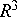Next: WIAS-Report-Serie Up: PreprintsReports Previous: PreprintsReports

## WIAS-Preprint-Serie

1. Anton Bovier, Véronique Gayrard, Pierre Picco: Gibbs states of the Hopfield model in the regime of perfect memory.
2. Roland Duduchava, Siegfried Prößdorf: On the approximation of singular integral equations by equations with smooth kernels.
3. Klaus Fleischmann, Jean--François Le Gall: A new approach to the single point catalytic super--Brownian motion.
4. Anton Bovier, Jean--Michel Ghez: Remarks on the spectral properties of tight binding and Kronig--Penney models with substitution sequences.
5. Klaus Matthes, Rainer Siegmund--Schultze, Anton Wakolbinger: Recurrence of ancestral lines and offspring trees in time stationary branching populations.
6. Karmeshu, Henri Schurz: Moment evolution of the outflow--rate from nonlinear conceptual reservoirs.
7. Wolfdietrich M uller, Klaus R. Schneider: Feedback stabilization of nonlinear discrete--time systems.
8. Gennadii A. Leonov: A method of constructing of dynamical systems with bounded nonperiodic trajectories.
9. Gennadii A. Leonov: Pendulum with positive and negative dry friction. Continuum of homoclinic orbits.
10. Reiner Lauterbach, Jan A. Sanders: Bifurcation analysis for spherically symmetric systems using invariant theory.
11. Milan Kucera: Stability of bifurcating periodic solutions of differential inequalities in.
12. Peter Knabner, Cornelius J. van Duijn, Sabine Hengst: An analysis of crystal dissolution fronts in flows through porous media Part I: Homogeneous charge distribution.
13. Werner Horn, Philippe Laurençot, J urgen Sprekels: Global solutions to a Penrose--Fife phase--field model under flux boundary conditions for the inverse temperature.
14. Oleg V. Lepskii, Vladimir G. Spokoiny: Local adaptivity to inhomogeneous smoothness. 1. Resolution level.
15. Wolfgang Wagner: A functional law of large numbers for Boltzmann type stochastic particle systems.
16. Hermann Haaf: Existence of periodic travelling waves to reaction--diffusion equations with excitable--oscillatory kinetics.
17. Anton Bovier, Véronique Gayrard, Pierre Picco: Large deviation principles for the Hopfield model and the Kac--Hopfield model.
18. Wolfgang Wagner: Approximation of the Boltzmann equation by discrete velocity models.
19. Anton Bovier, Véronique Gayrard, Pierre Picco: Gibbs states of the Hopfield model with extensively many patterns.
20. Lev D. Pustyl'nikov, J org Schmeling: On some estimations of Weyl sums.
21. Michael H. Neumann: Spectral density estimation via nonlinear wavelet methods for stationary non--Gaussian time series.
22. Karmeshu, Henri Schurz: Effects of distributed delays on the stability of structures under seismic excitation and multiplicative noise.
23. J org Schmeling: Estimates of Weyl sums over subsequences of natural numbers.
24. Grigori N. Milstein, Michael V. Tret'yakov: Mean--square approximation for stochastic differential equations with small noises.
25. Valentin Konakov: On convergence rates of suprema in the presence of non--negligible trends.
26. Pierluigi Colli, Jürgen Sprekels: On a Penrose--Fife model with zero interfacial energy leading to a phase--field system of relaxed Stefan type.
27. Anton Bovier: Self--averaging in a class of generalized Hopfield models.
28. Andreas Rathsfeld: A wavelet algorithm for the solution of the double layer potential equation over polygonal boundaries.
29. Michael H. Neumann: Bootstrap confidence bands in nonparametric regression.
30. Henri Schurz: Asymptotical mean square stability of an equilibrium point of some linear numerical solutions with multiplicative noise.
31. Gottfried Bruckner: On the stabilization of trigonometric collocation methods for a class of ill--posed first kind equations.
32. Wolfdietrich M uller: Asymptotische Input--Output--Linearisierung und St orgr oßenkompensation in nichtlinearen Reaktionssystemen.
33. Vladimir Maz'ya, Gunther Schmidt: On approximate approximations using Gaussian kernels.
34. Henri Schurz: A note on pathwise approximation of stationary Ornstein--Uhlenbeck processes with diagonalizable drift.
35. Peter Mathé: On the existence of unbiased Monte Carlo estimators.
36. Kathrin B uhring: A quadrature method for the hypersingular integral equation on an interval.
37. Gerhard H ackl, Klaus R. Schneider: Controllability near Takens--Bogdanov points.
38. Tatjana A. Averina, Sergey S. Artemiev, Henri Schurz: Simulation of stochastic auto--oscillating systems through variable stepsize algorithms with small noise.
39. Joachim F orste: Zum Einfluß der W armeleitung und der Ladungstr agerdiffusion auf das Verhalten eines Halbleiterlasers.
40. Herbert Gajewski, Konrad Gr oger: Reaction--diffusion processes of electrically charged species.
41. Johannes Elschner, Siegfried Pr ossdorf, Ian H. Sloan: The qualocation method for Symm's integral equation on a polygon.
42. Sergej Rjasanow, Wolfgang Wagner: A stochastic weighted particle method for the Boltzmann equation.
43. Ion G. Grama: On moderate deviations for martingales.
44. Klaus Fleischmann, Andreas Greven: Time--space analysis of the cluster--formation in interacting diffusions.
45. Grigori N. Milstein, Michael V. Tret'yakov: Weak approximation for stochastic differential equations with small noises.
46. G unter Albinus: Nonlinear Galerkin methods for evolution equations with Lipschitz continuous strongly monotone operators.
47. Andreas Rathsfeld: Error estimates and extrapolation for the numerical solution of Mellin convolution equations.
48. Mikhail S. Ermakov: On lower bounds of the moderate and Cramer type large deviation probabilities in statistical inference.
49. Pierluigi Colli, J urgen Sprekels: Stefan problems and the Penrose--Fife phase field model.
50. Mikhail S. Ermakov: On asymptotic minimaxity of Kolmogorov and omega--square tests.
51. Gunther Schmidt, Boris N. Khoromskij: Boundary integral equations for the biharmonic Dirichlet problem on nonsmooth domains.
52. Hans Babovsky: An inverse model problem in kinetic theory.
53. Dietmar H omberg: Irreversible phase transitions in steel.
54. Hans G unter Bothe: How 1--dimensional hyperbolic attractors determine their basins.
55. Ingo Bremer: Waveform iteration and one--sided Lipschitz conditions.
56. Herbert Gajewski, Klaus Zacharias: A mathematical model of emulsion polymerization.
57. J. Theodore Cox, Klaus Fleischmann, Andreas Greven: Comparison of interacting diffusions and an application to their ergodic theory.
58. Andreas Juhl: Secondary Euler characteristics of locally symmetric spaces. Results and Conjectures.
59. Nikolai N. Nefedov, Klaus R. Schneider, Andreas Schuppert: Jumping behavior in singularly perturbed systems modelling bimolecular reactions.
60. Roger Tribe, Wolfgang Wagner: Asymptotic properties of stochastic particle systems with Boltzmann type interaction.Next: WIAS-Report-Serie Up: PreprintsReports Previous: PreprintsReports

BREMERO
Wed Apr 12 21:47:02 MDT 1995